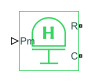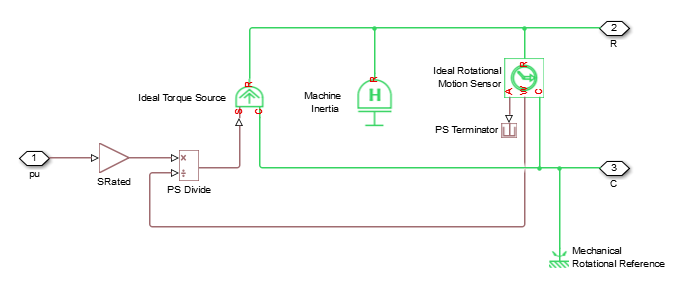# Machine Mechanical Power

Machine mechanical power defined in the SI or per-unit system

• Library:
• Simscape / Electrical / Electromechanical / Mechanical

•## Description

The Machine Mechanical Power block supplies specified power to, or draws specified power from, the machine that it connects to. It includes a representation of machine inertia and a mechanical rotational reference. In generator mode, the physical signal input defines the mechanical power that is input to the machine. In motor mode, it defines the mechanical power output from the machine.

The figure shows an equivalent configuration to the per-unit variant model of the Machine Mechanical Power block using Simscape™ mechanical rotational components.### Electrical Defining Equations

The SI model converts the SI values that you enter in the dialog box to per-unit values for simulation. For information on the relationship between SI and per-unit machine parameters, see Per-Unit Conversion for Machine Parameters. For information on per-unit parameterization, see Per-Unit System of Units.

To calculate the torque that it applies to the inertia, the block divides the power demand by the present speed. To set the peak torque limit, specify a value for the Peak torque to rated torque ratio parameter. Use the Specify inertia parameterization by parameter to specify inertia, J, directly or indirectly, with the inertia constant for the machine, H.

If you specify the inertia constant for the machine, the block calculates inertia as

`$J=\frac{2H{S}_{rated}}{{\left(2\pi {F}_{rated}/N\right)}^{2}},$`

where:

• J is inertia in kg⋅m2.

• H is the inertia constant in sW/VA.

• Srated is the rated apparent power of the connected machine in VA.

• Frated is the rated electrical frequency of the connected machine in Hz.

• N is the number of machine pole pairs.

Damping represents viscous friction between the machine rotor and mechanical rotational reference. Based on the value you select for the Specify damper parameterization by parameter, you specify a damping coefficient in per-unit or in SI units. If you specify the damping coefficient in per-unit, the block calculates the damping coefficient in SI units using these equations:

`${\omega }_{base}=\frac{2\pi {F}_{rated}}{N},$`

`${T}_{base}=\frac{{S}_{rated}}{{\omega }_{base}},$`

`${D}_{base}=\frac{{T}_{base}}{{\omega }_{base}},$`

and

`$D={D}_{pu}{D}_{base},$`

where:

• ωbase is the base mechanical speed in rad/s.

• Tbase is the base damping torque in Nm.

• Dbase is the base damping coefficient in Nm/(rad/s).

• Dpu is the damping coefficient in per-unit.

• D is the damping coefficient in SI units of Nm/(rad/s).

## Ports

### Input

expand all

Physical signal input port associated with mechanical power, in W.

#### Dependencies

This port is visible only if you set the PS input unit parameter in the Main setting to `SI`.

Physical signal input port associated with mechanical power, in per-unit.

#### Dependencies

This port is visible only if you set the PS input unit parameter in the Main setting to `Per unit`.

### Conserving

expand all

Mechanical rotational conserving port associated with the machine rotor.

Mechanical rotational conserving port associated with the machine case.

## Parameters

expand all

### Main

Machine type specification.

Rated apparent power of the connected machine.

Nominal electrical frequency corresponding to the rated apparent power of the connected machine.

Number of pole pairs of the connected machine.

Unit system for physical signal input.

Select `SI` to expose the Pm port or `Per unit` to expose the pu port.

Ratio that the block multiplies by the base torque to provide the upper limit for the torque that accelerates the inertia.

### Inertia

Inertia specification.

Inertia constant.

#### Dependencies

This parameter is visible only if you set the Specify inertia parameterization by parameter to `Inertia constant, H`.

Total rotational mechanical inertia of machine rotor. This parameter is visible only if you set Specify inertia parameterization by to ```Actual Inertia, J```.

#### Dependencies

This parameter is visible only if you set the Specify inertia parameterization by parameter to `Actual Inertia, J`.

Damping specification.

Damping coefficient in per-unit.

#### Dependencies

This parameter is visible only if you set the Specify damper parameterization by parameter to ```Per-unit damping coefficient, pu_D```.

Damping coefficient in SI units.

#### Dependencies

This parameter is visible only if you set the Specify damper parameterization by parameter to `SI damping coefficient, D`.

### Initial Conditions

Frequency initialization.

Initial electrical frequency.

#### Dependencies

This parameter is visible only if you set the Specify initialization by parameter to ```Initial electrical frequency```.

Initial mechanical frequency.

#### Dependencies

This parameter is visible only if you set the Specify initialization by parameter to ```Initial mechanical frequency```.

## Version History

Introduced in R2014b